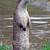This is a short program written to desmonstrate the integration of a potentiometer on the Gamebuino Meta. It is intended as a code example to show how to handle the hardware. The commented code is available on the discourse post related to this creation for everyone to use and discuss.Thomas Thuret
1 year ago

Here is the source code with comments. Feel free to ask questions about it and reuse it yourself.

``````#include <Gamebuino-Meta.h>
//we include the math library to get access to sin() and cos() functions as well as a value of PI used in the program
#include <math.h>

//variable declaration for the potentimeter value and its conversion to angle
int32_t i32_pot_value;
int32_t i32_pot_angle;

//variable declaration for the point used to draw a line
int16_t i16_point_x;
int16_t i16_point_y;

//define of the analog pin used to plug the potentiometer, here it's A1
#define ANALOG A1

//define of our circle properties, here it has a radius of 15 and is centered on the screen
#define CIRCLE_R 15
#define CIRCLE_X gb.display.width()/2
#define CIRCLE_Y gb.display.height()/2

void setup() {
// put your setup code here, to run once:
gb.begin();

}

void loop() {
// put your main code here, to run repeatedly:
while (!gb.update());

// the logic function will handle the math needed for the program
logic();

//the draw function will handle the display of all the elements needed for the program
draw();
}

void  logic(){
// we read the value from the potentiometer plugged to the analog port A1
// we convert the value from a range of 0 to 1023 to an angle within 360 degrees
i32_pot_angle = (i32_pot_value * 360) / 1023;

// we calculate the coordinates of a point on the circle perimeter corresponding to the value of the angle found above
// note the multiplication by PI/180, this is because the angle we calculated in degrees must be converted to radians
i16_point_x = CIRCLE_X + CIRCLE_R * cos(i32_pot_angle * M_PI/180);
i16_point_y = CIRCLE_Y + CIRCLE_R * sin(i32_pot_angle * M_PI/180);
}

void  draw(){
// the clear function is needed to reset the screen between each frame, otherwise frames would mix together and get messy
gb.display.clear();

// we print the value read from the potentiometer and its value when converted to an angle
gb.display.print(" pot_value = ");
gb.display.print(i32_pot_value);
gb.display.print("\n angle = ");
gb.display.print(i32_pot_angle);

// we draw the circle with the properties we defined at the beginning, its center's position and radius
gb.display.drawCircle(CIRCLE_X, CIRCLE_Y, CIRCLE_R);
// we draw a line between the center of the circle and the point on its perimeter corresponding to the potentiometer value we read
gb.display.drawLine(CIRCLE_X, CIRCLE_Y, i16_point_x, i16_point_y);
}

// code by Thomas Thuret for Gamebuino
``````